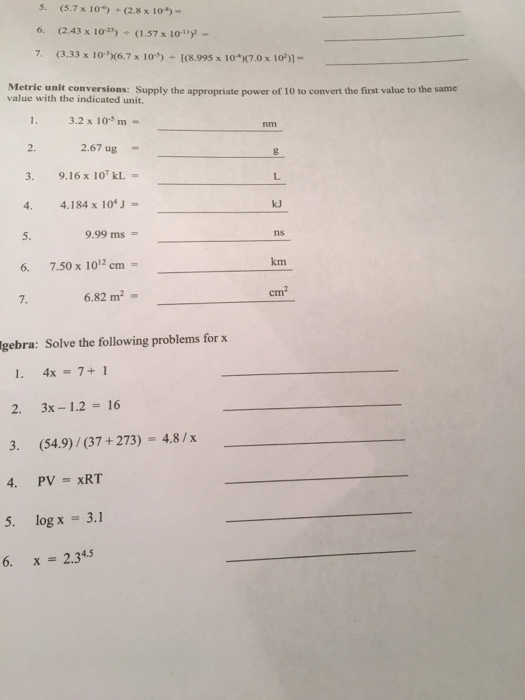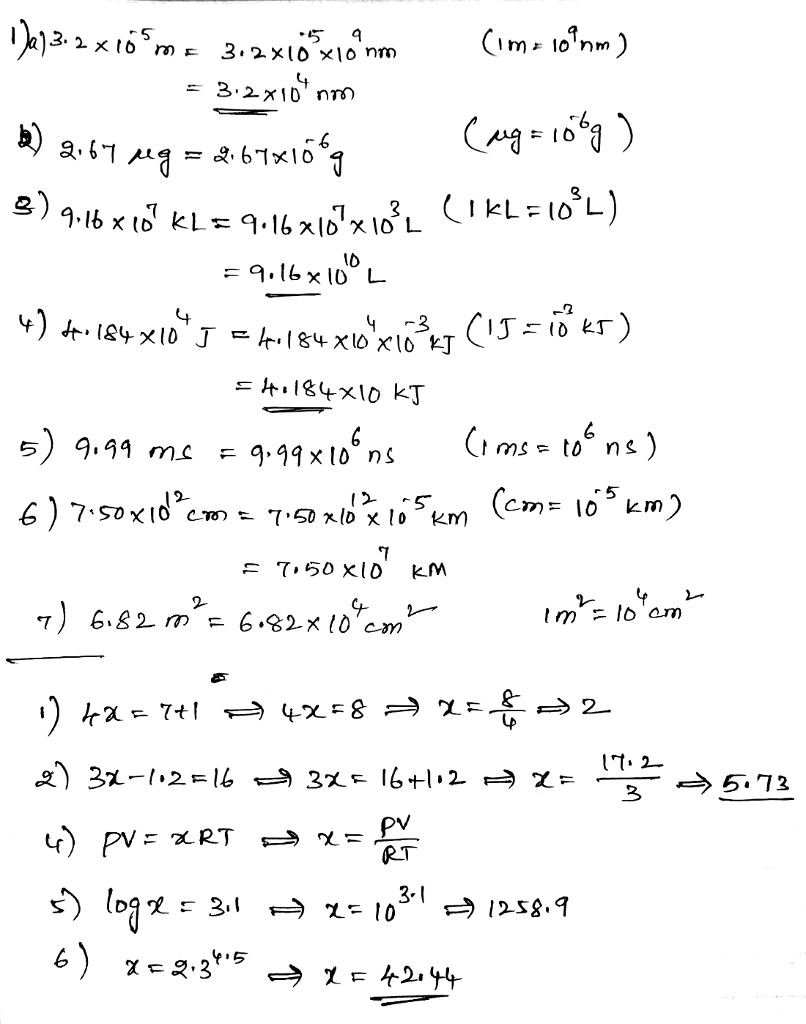# Homework Solution: Supply the appropriate power of 10 to convert the first value to the same value…Supply the appropriate power of 10 to convert the first value to the same value with the indicated unit. 3.2 times 10^-5 m = _____nm 2.67 ug = _____g 9.16 times 10^7 kL = _____L 4.184 times 10^-4 J = _____kJ 9.99 ms = _____ns 7.50 times 10^12 cm = ____km 6.82 m^2 = _____cm^2 Solve the following problems for x 4x = 7 + 1 ____ 3x - 1.2 = 16 _____ (54.9)/(37 + 273) = 4.8/x _____ PV = xRT ____ log x = 3.1 _____ x = 2.3^4.5 _____________